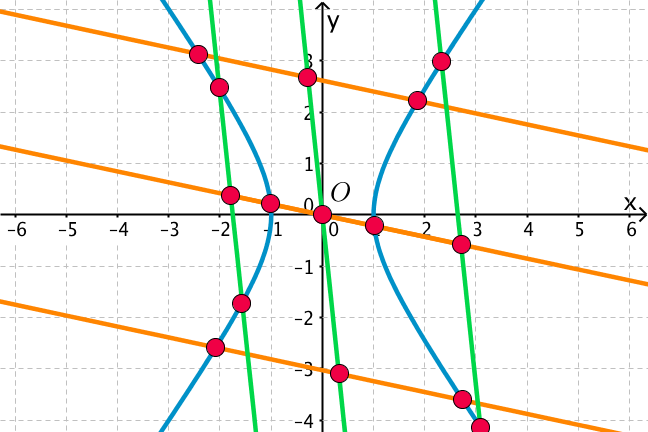## Want to keep learning?

This content is taken from the UNSW Sydney's online course, Maths for Humans: Inverse Relations and Power Laws. Join the course to learn more.
1.4

## UNSW SydneyA classical property of a hyperbola

# Classical properties of hyperbolas

Hyperbolas are special kinds of conic sections and were studied extensively by Apollonius of Perga around 200 B.C. In some ways they are analogous to ellipses, but they also have some remarkable additional features.

In this step we will

• learn about the asymptotes, tangent lines and reflective properties of hyperbolas

• discuss the focus and directrix definition of a hyperbola

## Hyperbolas, cones and asymptotes

Apollonius thought of hyperbolas as being slices of a cone, extending indefinitely in both directions, with a plane that cuts both parts of the cone. One of the key properties of such a hyperbola is the existence of two asymptotes: lines which the hyperbola does not meet but which it approaches closer and closer as we move further outwards in the plane.

Geometrically, the asymptotes are the lines which form the boundary of the perpendicular projection of the cone onto the plane. Neither ellipses nor parabolas have asymptotes, and this makes the geometry of the hyperbola somewhat special. The hyperbola also has a centre which is a point of symmetry. The centre is the meet of the two asymptotes.## The eccentricity of a hyperbola

A hyperbola may also be defined in terms of a focus $$\normalsize{F}$$ and a directrix $$\normalsize{l}$$, such that for any point $$\normalsize X$$ on the hyperbola,

$\Large{\frac{|X,F|}{|X,l|}=e>1}$

for some fixed constant $$\normalsize{e}$$ called the eccentricity of the hyperbola. This condition can also be restated in terms of quadrances, which makes it more algebraic and suitable for calculation:

$\Large{\frac{Q(X,F)}{Q(X,l)}=e^2>1}.$

We remind you that the quadrance between points is just the square of the distance between those points, and that the quadrance from a point $$\normalsize{X}$$ to a line $$\normalsize{l}$$ means the quadrance from $$\normalsize{X}$$ to the closest point $$\normalsize{P}$$ on the line. Quadrance is often easier to work with than distance because no messy square roots are involved, and it also forms the basis of the new theory of Rational Trigonometry, which is very exciting.## Calculating the equation of a hyperbola

Let’s use the above characterisation of a hyperbola with focus $$\normalsize{F=[2,0]}$$, directrix the line $$\normalsize{x=1}$$ and eccentricity $$\normalsize{e=2}$$ to find its equation. Using the quadrance form, if $$\normalsize{X=[x,y]}$$ is an arbitrary point on the hyperbola, then

$\Large{Q(X,F)=(x-2)^2+y^2}$

and

$\Large{Q(X,l)=(x-1)^2}$

so the equation is

$\Large{\frac{(x-2)^2+y^2}{(x-1)^2}=2^2}.$

We rewrite this as

$\Large{(x-2)^2+y^2=4(x-1)^2}$

which simplifies (please check the algebra!) to

$\Large{3x^2-y^2-4x=0}.$

Q1 (E): Find the equation of the hyperbola with focus the point $$\normalsize{F=[0,2]},$$ directrix the line $$\normalsize{y=1}$$, and eccentricity $$\normalsize{e=2}$$.

In fact there are always two pairs of foci and directrices for a hyperbola. We can see this since the reflection in the centre of the hyperbola will take one pair to the other.

Q2 (M): What is the centre of the hyperbola that you found in Q1?

Q3 (C): What is the other focus, and what is the other directrix of the hyperbola in Q1?

## Reflective property

Another property of the hyperbola is that if a light ray is emitted from one focus $$\normalsize{F_1}$$, then it reflects off the hyperbola along a line which passes through the second focus $$\normalsize{F_2}$$.This property also holds for an ellipse, but in the case of the hyperbola the reflected ray moves away from the second focus while for an ellipse it moves towards it.

For an ellipse, this property is responsible for the interesting phenomenon of whispering rooms: if a room has a ceiling with an ellipsoidal shape, then someone standing at one focus can whisper to a friend standing at a second focus and they can hear, since the sound waves bounce off the ceiling and all converge to the other focus. A famous example occurs in St. Paul’s cathedral in London.St Paul’s Cathedral Whispering Gallery DeZeeuw from nl GFDL or CC-BY-SA-3.0, via Wikimedia Commons

## Tangent property of a hyperbola

A property of the hyperbola discovered by Apollonius states that for a line tangent to the hyperbola at a point $$\normalsize{X}$$ and intersecting the asymptotes at points $$\normalsize{P}$$ and $$\normalsize{R}$$, the product $$\normalsize{\vert O,P\vert \times \vert O,R\vert}$$ is constant, and $$\normalsize{\vert P,X\vert=\vert R, X\vert}$$.

Of course it is much more pleasant if we restate these conditions rationally as

$\Large Q(O,P)\times Q(O,R)= \text{constant}$

and

$\Large Q(P,X)=Q(R,X).$Q4 (C): Consider the initial image for this step. It also shows a property of the hyperbola. Can you deduce what it is?

A1. Using the quadrance form, if $$\normalsize{X=[x,y]}$$ is an arbitrary point on the hyperbola, then

$\Large{Q(X,F)=x^2+(y-2)^2}$

and

$\Large{Q(X,l)=(y-1)^2}$

so the equation is

$\Large{\frac{x^2+(y-2)^2}{(y-1)^2}=2^2}.$

We rewrite this as

$\Large{x^2+(y-2)^2=4(y-1)^2}$

which simplifies to

$\Large{x^2-3y^2+4y=0}.$

A2. Let’s complete the square to rewrite the hyperbola $$\normalsize{x^2-3y^2+4y=0}$$ as

$\Large{(x+0)^2-3(y-2/3)^2=-\frac{4}{3}}.$

Since the centre of the hyperbola $$\normalsize{x^2-3y^2=-\frac{4}{3}}$$ is the origin $$\normalsize{[0, 0]}$$, the centre of the hyperbola $$\normalsize{x^2-3y^2+4y=0}$$ will be the point $$\normalsize{[0, 2/3]}$$.

A3. To get the second focus and directrix, we reflect the first focus and directrix in the centre $$\normalsize{[0, 2/3]}$$. The second focus will be the point $$\normalsize{[0, -2/3]}$$, and the second directrix will be the line $$\normalsize{y=1/3}$$.

A4. The initial image for this step shows conjugate diameters for a hyperbola. That is something we discussed in our earlier course Maths for Humans: Linear and Quadratic Relations. The idea is that if you look at chords parallel to a given diameter, that is a chord through the centre, and take midpoints of those chords, then you get another diameter. And if you apply the same trick to this new diameter, you get back the diameter you started with.